# Designing and Building Real DIY Softboxes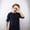Professional softboxes cost hundreds of dollars, and the cheap eBay ones are a risky investment in terms of quality. The DIY route may seem daunting, but this guide should make designing and building your own custom softbox feel within your grasp. Well, that is if you're not afraid of a little math!

## Introduction

With the scope of this article, I'm assuming you know what a softbox is and why you need it (unless you're a magical unicorn of light like Joe McNally).

We've all seen the before-and-after photos illustrating why you need a softbox, and now you want to take that next step. But putting up the kind of cash they seem to demand is either impossible, daunting, or outright offensive to you. So what do you do? You build your own, of course!

I'm going to cover a variety of shapes and sizes of reflector, as each requires a slightly different method for designing and some have particular concerns or uses to be aware of.

In this theoretical primer, by the end you should understand the factors which contribute to the quality of the light from a softbox, how the light moves through the softbox, and how to set about designing your own to build.

I'm going to keep the mathematics as basic as possible, and accompany it with as many diagrams as possible to help keep it relatable to physical phenomena, but if you can roughly remember your high school geometry lesson, you should find it reasonably straightforward.

## Pyramid Reflectors

The first type of softbox I'm going to look at is the usual kind that people tend to make themselves, the flat-sided, pyramidal shape. There's even a tutorial on this very site to help you with the build here.

These are very easy to construct and require very little math to design. The downside of this ease of construction is the fact that the light quality they produce isn't as high as from a parabolic reflector. This is due to the fact that the sides are the same angle all the way up, but of course the light is from a single point (the strobe head) so it's not hitting all of the wall at the same angle and being bounced out straight at the subject.

It's more spread out, similar to a strobe head itself (Fig. 1). Good diffusion material at the front will help alleviate this issue a little though. However, if all you're looking for is a simple build in the size and shape that you want as a start to your foray into light modification, this type should do the trick.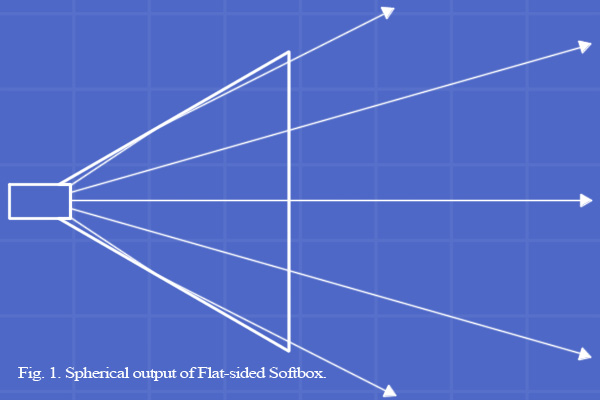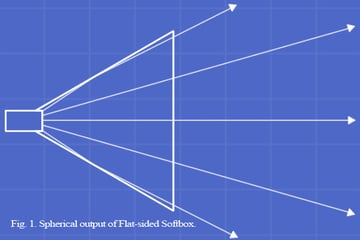Fig. 1: The spherical output of flat-sided softbox.

## The Simplicity of Pyramids

For pyramidal construction based on a regular polygon being symmetrical, with all equal sides, all you need is a set of identical isosceles triangles which attach together at the equal sides. To create these triangles you need just two measurements, based on your desired dimensions.

Using some basic trigonometry, we can convert these three-dimensional product dimensions (Fig. 2) into two-dimensional net dimensions to cut out (Fig. 3). Different numbers of sides require slightly different equations, so here they are for four-, six-, and eight-sided reflectors. Once you see how it works, you can do different numbers of sides with ease.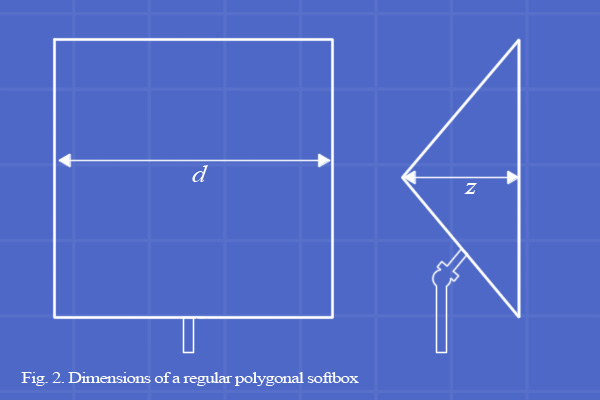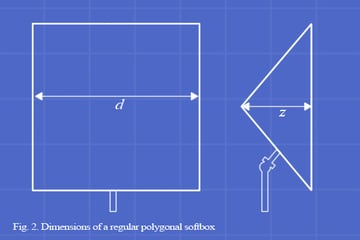Fig. 2: Dimensions of a regular polygonal softbox.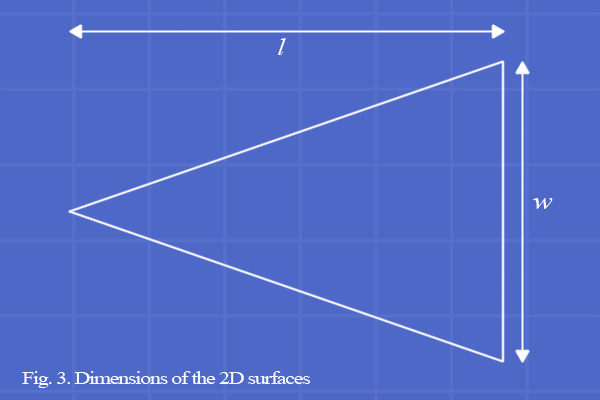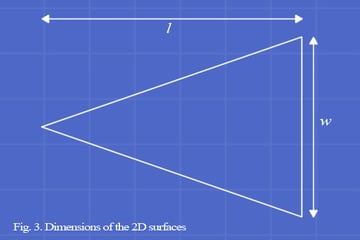Fig. 3: Dimensions of the 2D surfaces.

## Square Pyramid

Fig. 4 shows the two right-angle triangles within a pyramid which we can use some basic trigonometry on to calculate the l and w values we need.

You'll note that in the case of square pyramids, softbox diameter d and triangle width w are equal, so we don't need to use the red triangle in this instance. We do, however, need triangle length l because of course, it's not the same as softbox depth z. To find l based on z and d, we can refer to good old Pythagorean Theorem: a2 + b2 = c2.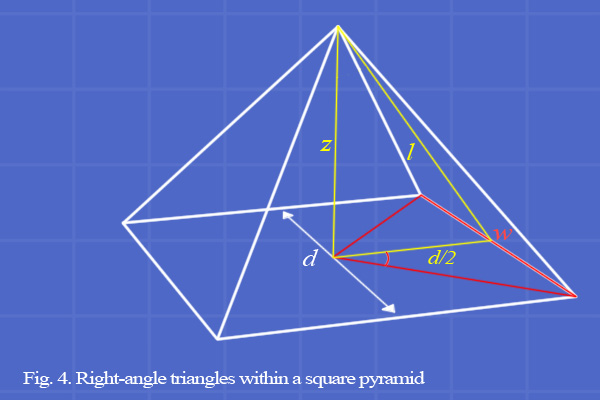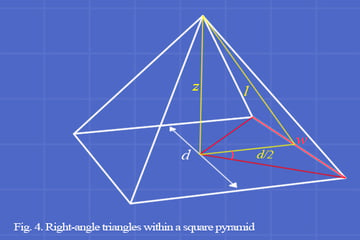Fig. 4: Right-angle triangles within a square pyramid.

## Pythagoras

The variable c refers to the longest side of the triangle, the hypotenuse. This is our l. The other two sides which form the right angle are a and b, in no particular order.

Clearly one of these is z, and it can be seen from Fig. 4 that the final side is half of the diameter of the softbox, d/2. Since we know the length of both z and d (that is, you've already decided on them based on the size of softbox you need, the size of your studio, the amount of material you have to build with, or whatever parameters you use to decide), we can apply Pythagoras to them as follows in Fig. 5.

For this example, I've used 47cm (the depth of the ProFoto softboxes) for z and 90cm for d, to make a reasonably deep three foot square softbox: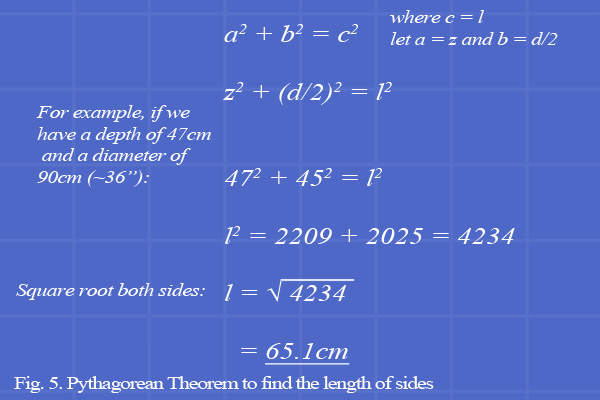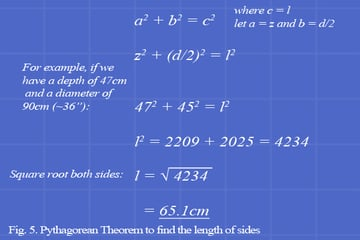Fig. 5: Pythagorean Theorem to find the length of sides

Once you have your l and w values, simply draw out a triangle with them as shown in Fig. 3, and use this as your template to cut out 4 identical triangles.

## Hexagonal Pyramid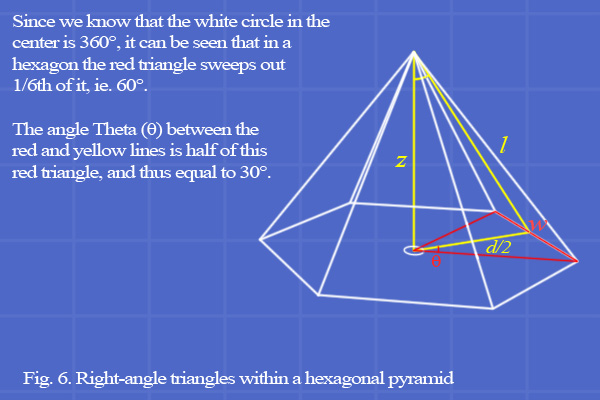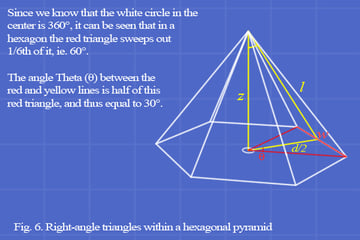Right-angle triangles within a hexagonal pyramid.

This is a similar setup, and finding l is, as you can see, the same as in Fig. 5. However, since w is no longer equal to the diameter of the softbox, we need another way of finding it. This is where the red triangle comes in, and a new piece of basic trigonometry.

If you recall the mnemonic SOHCAHTOA from high school, you're already half way there! The part of it we'll be using is the TOA at the end, which is the short form of remembering that the tangent of an angle is equal to the opposite side length divided by the adjacent side length.

Since the yellow line is half of the diameter (ie. d/2), which equals the radius r, and the angle θ is 30°, we can do as follows.

## Trigonometry 101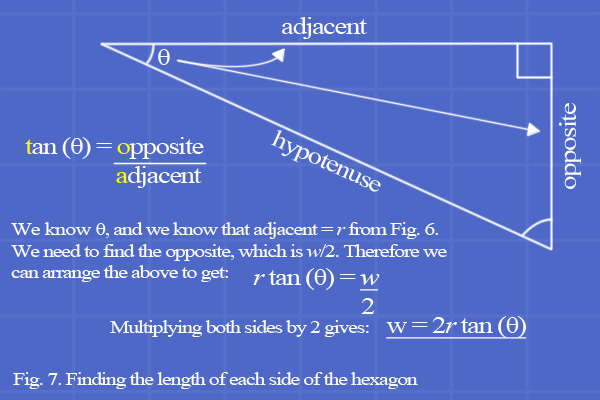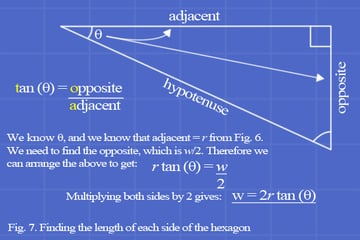Finding the length of each side of the hexagon

A quick example: if the diameter of your softbox is again 90cm, the radius would be 45cm. The equation in Fig. 7 then becomes: w = (2*45) tan (30), which is equal to 52.0cm.

## Octagonal Pyramid

An octagonal pyramid is, practically speaking, no different to the hexagonal pyramid. The only thing you need to calculate differently is w, since with 8 sides, the "red triangle" is 360°/8 = 45°. Half this to find the angle we need and we have θ = 22.5°.

## Practical Considerations

The main construction material I had in mind when writing this article was normal corrugated cardboard, but other materials like heavy paper or card, fluted/corrugated plastic (Correx), or similar could also be used depending on the size and weight requirements of your design.

Unless you make your template out of plastic or similar, I would recommend just using it to mark off the corners of the shape on the cloth and not drawing around it tightly. This would cause it to fray and your sectors will become less accurate with each drawing. If you only mark the corners, you can then just link them up with a ruler afterward and it would be much more consistently accurate.

## That's It For Pyramids

That's the end of the pyramid section. As you can see, while the math might look daunting at first, they're really very easy to design and build, ultimately only needing the two simple calculations I've given here.

Now you can design basic pyramidal softboxes in any shape and size you like! Once you've grasped the math, you should be able to expand it further yourself into rectangular softboxes, conical ringflashes, or even more.

In part two I'll be covering parabolic reflectors in detail. Since they're the best shape of reflector, if you're more technically able and just want the best light possible, make sure to tune in for that!

Don't forget, if you have any questions or comments hit up the comments section below.

## Parabolic Reflectors

Next, I'll be covering parabolic reflectors, what they are, how they work, and how to design your own. There'll also be some more practical pointers at the end to help your build.

I've made a simple one-page PDF to help anyone who's having trouble wrapping their head around the theory and would like a physical model to help. Just cut it out, tape it together, and compare the numbers on the model to the numbers in the table. It should help explain matters. Even if you're fine with the math, perhaps you'd like to test the concept small-scale before you invest more time and resources.

## What is a Parabola, Exactly?

A paraboloid is a 3D shape formed by the rotation of a parabola, a curve based on a quadratic formula (i.e. y = mx2). A parabola with a focal length f has an m value of 1/4f.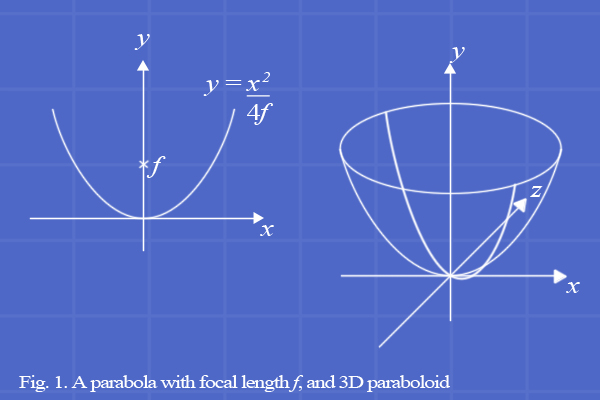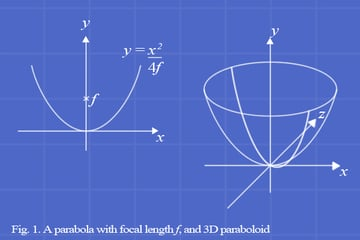A parabola with focal length f, and 3D paraboloid.

If the interior surface is reflective, parabolas have an optical quality of sorts, somewhat like inverted lenses.

## Optical Properties

As shown in Fig. 2, the depth of a parabola affects its focal length. This is related to a piece of advice you may have heard previously: "deeper softboxes produce better light." Why is this? Well, it's related to the distance between the flash head and the focal length.

While shallow parabolas with long focal lengths may work for bounce umbrellas where the flash is a distance away in front of it, in softboxes the flash is at or near the back. How does this movement affect the quality of light? Here's an illustration of the effect: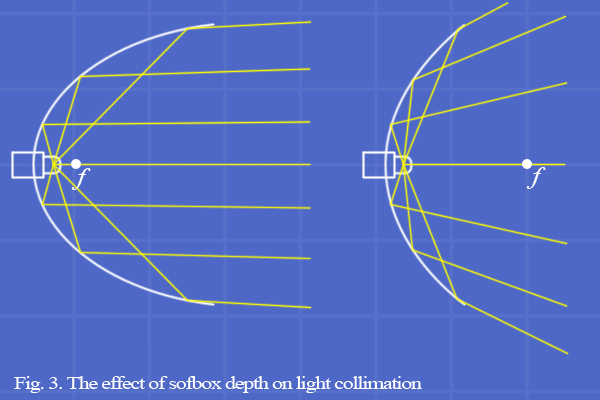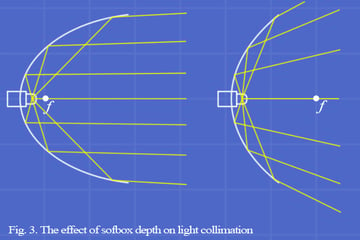The effect of sofbox depth on light collimation.

## What Does This Mean For The Light Quality?

Clearly, there's more to a softbox than simply bouncing light forward and diffusing it. The issue is that "soft light" isn't simply a product of diffusion, but collimation. That "wrapping" effect that portrait photographers love so much doesn't come simply from firing a strobe into a diffusion cloth, but from having a beam of light with rays as parallel as possible (collimation) from a source wider than the subject.

Diffusing these parallel rays then causes the wrap. Essentially, the idea is to replicate a cloudy sky. As can be seen in image above, the shallow parabola with the long focal length produces a much wider spread of light. While this is useful in increasing the power of the strobe by directing more light forwards, the result is that the actual shape of the light propagation is little different to a bare flash head.

This causes the "hotspot" effect, and doesn't tend to result in the pleasing light wrapping effect as strongly. Shallowness is relative, of course. A 2ft softbox that's 47cm deep is really deep. But a 6ft softbox of the same depth is relatively much shallower.

It's possible to reduce this effect in wide softboxes, though, with the use of an interior light baffle. This is simply a second diffuser which fits inside the softbox at about half the depth. It diffuses the light out from the centre, eliminating that hotspot, and at the same time pushing more light in a generally forward direction: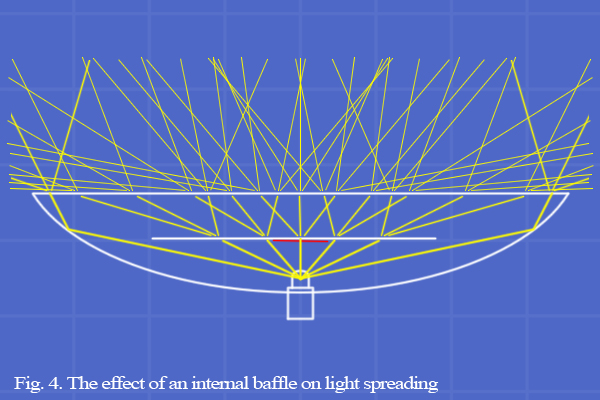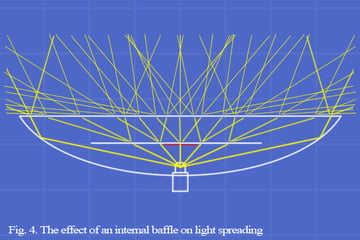The effect of an internal baffle on light spreading.

## Internals

In this illustrative diagram, I've only drawn the transmissive rays. The baffle has the secondary effect of reflecting light back onto the parabolic reflector from a wide angle at close range, giving the effect of a point light source further away from the reflector.

This creates a set of light rays that become more collimated, enhancing the wrapping effect. Some internal baffles even have silvered circles in the center (shown here in red) to cut out the hotspot altogether and further spread out the light across the rear reflector.

## Making Softboxes

Now that you've transported back to high school math class, let's put some rubber to the road. This process may seem complex, but in practice it isn't significantly different to building a simple pyramid reflector, but more like making many slices of pyramids of varying diameter and depth, like thus: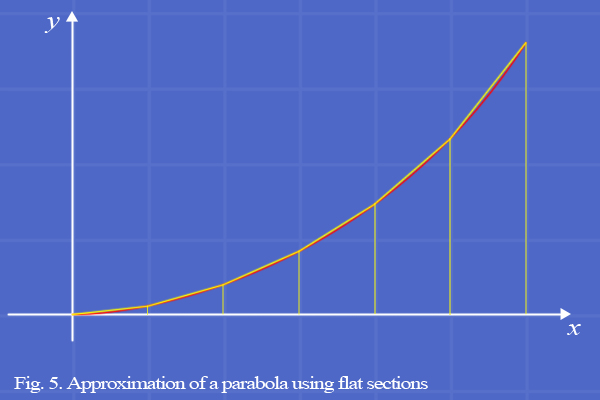Approximation of a parabola using flat sections.

## Section Lengths

I'm using six sections throughout for clarity, but it would probably be better to use closer to ten sections for optimized light shaping. As you can see, the length of each individual section increases as it gets closer to the outer edge. So first we must calculate these changing lengths, which I've termed dl.

Since we know the x-value of all of the points, as in the figure below, and the equation of the curve (given your chosen f-value) we can use this to calculate the length of each section, dl.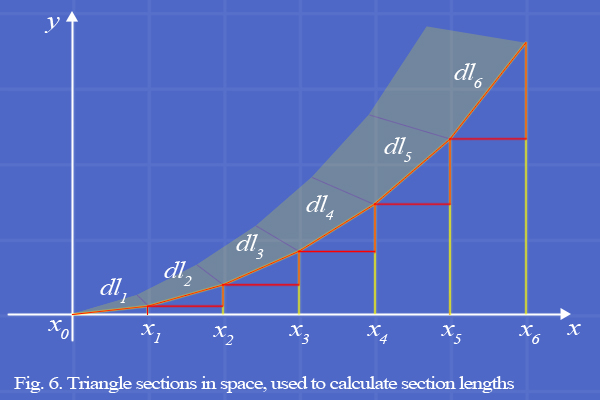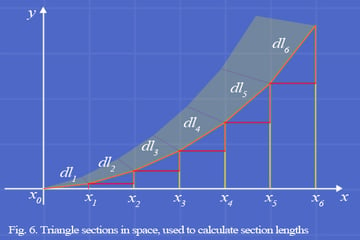Triangle sections in space, used to calculate section lengths.

Note that the difference between the current and previous x-values form the base of a triangle, and between the current and previous y-values form the side. Given this knowledge, it's easy to find the hypotenuse dl by simply using Pythagoras in the same way as we did on the pyramidal softbox.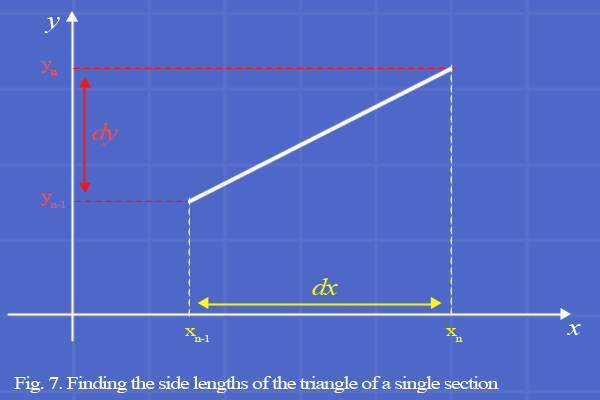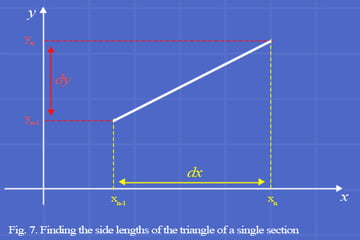Finding the side lengths of the triangle of a single section.

Since the x-values are a regular distance apart, dx takes the value of this distance for the whole range. Then we simply move through the y-values, subtracting the previous value from the current value to get a range of dy values. Using Pythagorean Theorem on each of these in turn will yield our set of dl values.

As an example, I'll return to my previous parameters of 90cm diameter and 47cm depth. Given a 45cm radius, I'll use just 5 sections of 9cm for this example. In practice, I would likely use 9 sections of 5cm for this particular size softbox.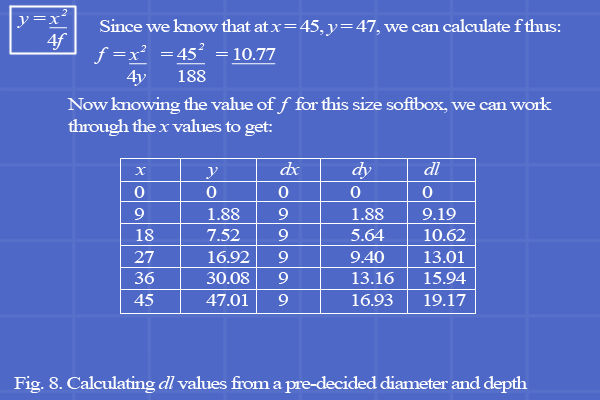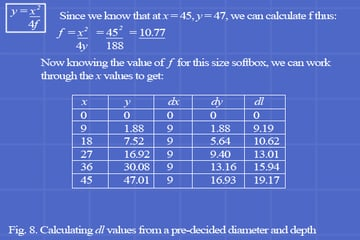Calculating dl values from a pre-decided diameter and depth

Naturally, y is simply calculated using the equation of a parabola, in the top left corner. If you look closely, the dy values are the current y value minus the previous y value as I described above.

## Section Widths

Now we have our lengths of our sections, all that's left to find is our widths. Depending on whether it's a square or an octagon, there are two possible methods of doing this. For a square, simply double the current x value. Easy!

For an octagon, though, it's the same as the octagonal pyramid, w = 2r tan (θ) but at each value of x. Remember to use the x values for r and not the dl values! You want it to fit together once assembled in shape, not when it's still flat!

If you're confused as to why the first value of everything is always zero, it starts at a point with no length or width, and has zero distance since the previous point because there is no previous point: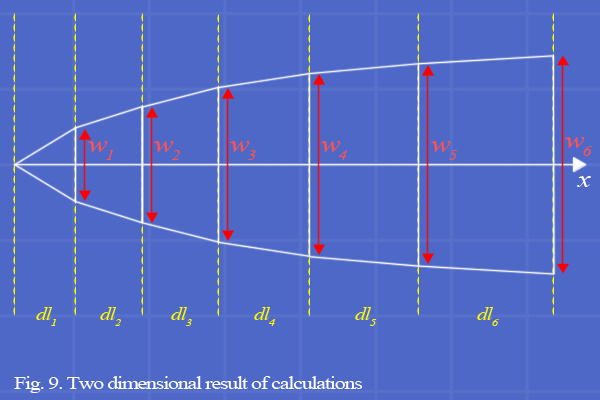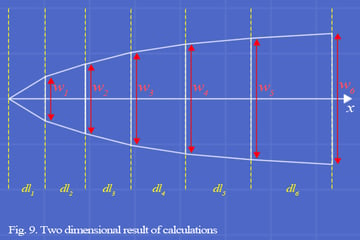Two dimensional result of calculations.

Once you're done, you should have several sets of numbers that look roughly like the above table (with w values too, of course), which, when plotted out onto paper look approximately like the image above.

The angle of the sides should always be decreasing towards the outside- remember that tubes have parallel walls and flat shapes have strongly angled sides. The parabola should become more tubular as it gets closer to the outside.

Now, if you're thinking all of that seems like way too much work and you might just stick with a pyramid softbox or take a chance on a cheapish one on eBay, take heart!

I've written a calculator in Javascript for you all to use to design parabolic reflectors in both square and octagonal shapes, of any size you like. It can be found secreted away on my website here: http://robtaylorcase.com/Calc/para. I've included all of the script within the webpage itself, so feel free to download the page to use offline or mirror it on your own site.

## Mounting the Flash

Since the scope of this article is primarily concerning the construction of the reflector itself, I'm just going to scratch the surface of a few other elements you should bear in mind.

Attaching the flash unit can be done in a variety of ways. If you're building a parabolic reflector, you should be trying to get the head as close to the focal point in order to maximize the effectiveness of the shape at collimation.

If you're not limited by the dimensions or availability of your construction material, you could try a few different depth values and see if you can get f within a few centimeters of the back of your reflector. This will make mounting your flash more convenient and maximize the quality of light you'll get from your softbox.

You could go the way as shown the PhotoTuts+ tutorial I linked to at the beginning and use the reflector material itself to hold the flash in place. Attaching this setup to a stand or tripod may be something of a challenge, however. That tutorial seems to go the most sensible route by attaching a bracket below the softbox's center of gravity and using this to slot into a swivel hotshoe mount.

You could alternatively cut a hole in the back of the reflector and bolt on a speedring and mounting bracket. While speedrings are relatively costly at $30 even for the cheap ones on eBay, this method would be the most convenient as the metal ring will hold the softbox securely while allowing for rapid movement of all parts as needed. And, if you upgrade to studio strobes, this means the mounting system required for those is already in place. You could try a DIY speedring method by taking a 6" or 8" shallow pizza pan or similar which you should be able to get for$2 or less, Dremelling a nice big hole in the middle so you have a roughly 3 to 4cm thick ring, and drilling holes evenly spaced around that ring to bolt it to your reflector, and to bolt on a right angle bracket with 1/4" holes which mounts your flash and connects to your stand/tripod.

## Rectangular/Oblong Softboxes

For non-square softboxes, for example 36x24" panels or 1x3ft striplights, make the reflector sectors the same, but then cut two of them in half lengthways from the point. Between the two pieces of each sectors, attach a rectangle of material as desired.

Use these rectangular pieces opposite each other in the three-dimensional product. These rectangular parts will have to be scored lengthways into sections to fit to the main parabolic sectors. To find the width of each section, measure the edge lengths of the parabolic sections the rectangle will fit to. These edges will not be the same as the dl values.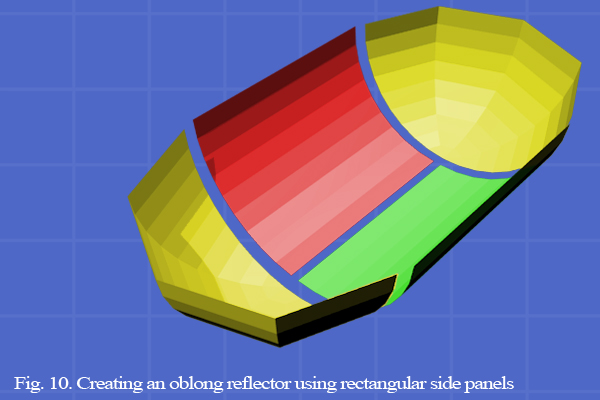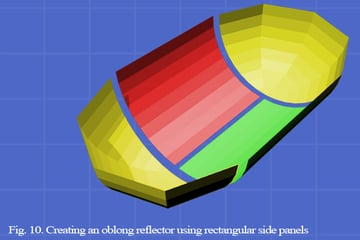Creating an oblong reflector using rectangular side panels.

You could, if you want a perfect paraboloid, use the calculator and mark out your points as normal, but instead of using that as-is, scan it into Photoshop and draw vector curves which pass perfectly through each point. Then blow this up, print it and laminate it for edge strength, and you have a template for reproducing perfect parabolas every time. Alternatively you could even graph the points in Excel with a smooth curve, print it and convert that to vector in Photoshop.

## Enjoy It!

The ideas in this article could easily be applied to other types of light modifier like beauty dishes and ringlights. The mathematics of reflectors applies universally.

And that's (finally) it! I hope you've learnt something from this article, don't worry if you have to reread it a few times, get a mathematically-inclined friend to help, or test your understanding with small-scale paper models first, and most of all I hope that you gain the confidence to build the softbox that you've always wanted to have!

Happy designing! If you have any questions or comments hit up the comments section below.